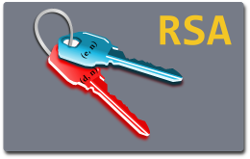## RSA Encryption and Signature Lab

### OverviewRSA (Rivest-Shamir-Adleman) is one of the first public-key cryptosystems and is widely used for secure communication. The RSA algorithm first generates two large random prime numbers, and then use them to generate public and private key pairs, which can be used to do encryption, decryption, digital signature generation, and digital signature verification. The RSA algorithm is built upon number theories, and it can be quite easily implemented with the support of libraries.

The learning objective of this lab is for students to gain hands-on experiences on the RSA algorithm. From lectures, students should have learned the theoretic part of the RSA algorithm, so they know how to generate public and private keys and can conduct operations, such as encryption, decryption, signature generation, and signature verification. This lab enhances student's learning by requiring students to conduct RSA operations on actual numbers, and see whether they can get the calculations right. This way, they are given a chance to apply the RSA theories learned from the class.